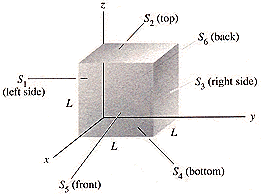# Electric Flux Through a Cube

## Homework Statement

A cube has sides of length L = 0.500 m. It is placed with one corner at the origin as shown in Fig. 23-29. The electric field is uniform and given by E = (2.50 N/C) i - (4.40 N/C) j.Find the electric flux through each of the six cube faces S1, S2, S3, S4, S5, and S6.
Find the electric flux through the entire cube.

## Homework Equations

$$\phi$$=EAcos$$\theta$$
$$\phi$$=q/$$\epsilon$$

## The Attempt at a Solution

I tried solving for the area of each side and multiply it by the electric field (using the pythagoreon theorem to get it) but that's gotten be nowhere.

For the first part, remember what the dot product means. You multiply the parts of the vectors that are parallel.
With that in mind, take mind of the sign convention. The area vector always points away from the enclosed volume.

As for the second part of the question, use Gauss' Law to avoid adding many terms together. ;)

For the first part, remember what the dot product means. You multiply the parts of the vectors that are parallel.
With that in mind, take mind of the sign convention. The area vector always points away from the enclosed volume.

As for the second part of the question, use Gauss' Law to avoid adding many terms together. ;)

What do you mean about the vector part?
Since the i is positive and the j is negative, does that mean it's pointing down and out on the x axis?

(And we're just learning Gauss' Law, still trying to understand it)

What do you mean about the vector part?
Since the i is positive and the j is negative, does that mean it's pointing down and out on the x axis?

(And we're just learning Gauss' Law, still trying to understand it)

That means that there are two electric fields. One that's in the $$+\hat i$$ direction, and one that's in the $$-\hat j$$ direction

Taking the dot product over any one side, you'll see that the component of the E-Field that's parallel to the surface (Perpendicular to the Area Vector) does not contribute at all to the dot product (And therefore, does not contribute to the flux).

As for Gauss' Law:

The total flux of the E-Field through any enclosed surface is equal to the total charge enclosed by the surface divided by the dielectric constant.

In integral notation:

$$\oint \vec E \cdot \vec {dA} = \frac{Q_{enclosed}}{\epsilon_0}$$